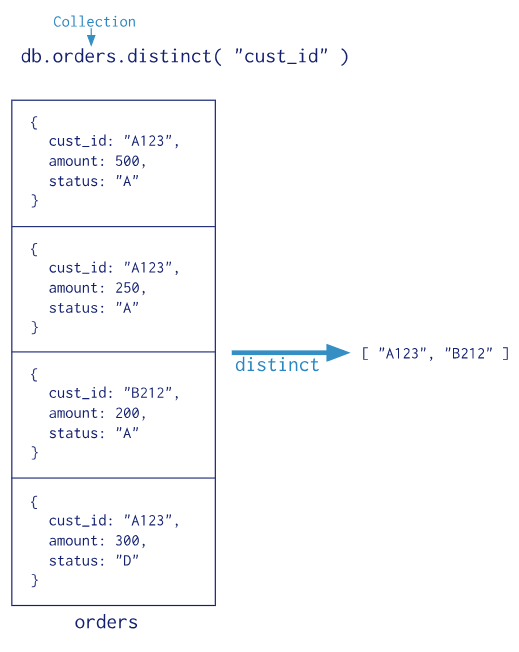# 一、基本概念

MongoDB中聚合(aggregate)主要用于处理数据(诸如统计平均值,求和等)，并返回计算后的数据结果。有点类似sql语句中的 count(*)。

## 1.1、aggregate() 方法

MongoDB中聚合的方法使用aggregate()。

### 语法：

>db.COLLECTION_NAME.aggregate(AGGREGATE_OPERATION)


## 1.2、实例

{
title: 'MongoDB Overview',
description: 'MongoDB is no sql database',
by_user: 'w3cschool.cc',
url: 'http://www.w3cschool.cc',
tags: ['mongodb', 'database', 'NoSQL'],
likes: 100
},
{
title: 'NoSQL Overview',
description: 'No sql database is very fast',
by_user: 'w3cschool.cc',
url: 'http://www.w3cschool.cc',
tags: ['mongodb', 'database', 'NoSQL'],
likes: 10
},
{
title: 'Neo4j Overview',
description: 'Neo4j is no sql database',
by_user: 'Neo4j',
url: 'http://www.neo4j.com',
tags: ['neo4j', 'database', 'NoSQL'],
likes: 750
}


> db.mycol.aggregate([{$group : {_id : "$by_user", num_tutorial : {$sum : 1}}}]) //结果 {"_id" : "w3cschool.cc", "num_tutorial" : 2 } {"_id" : "Neo4j","num_tutorial" : 1 }  以上实例类似sql语句：  select by_user, count(*) from mycol group by by_user  在上面的例子中，我们通过字段by_user字段对数据进行分组，并计算by_user字段相同值的总和。 # 二、常用聚合表达式 表达式 描述 实例$sum 计算总和。 db.mycol.aggregate([{$group : {_id : "$by_user", num_tutorial : { $sum : "$likes"}}}])
$avg 计算平均值 db.mycol.aggregate([{$group : {_id : " $by_user", num_tutorial : {$avg : " $likes"}}}])$min 获取集合中所有文档对应值得最小值。 db.mycol.aggregate([{ $group : {_id : "$by_user", num_tutorial : { $min : "$likes"}}}])
$max 获取集合中所有文档对应值得最大值。 db.mycol.aggregate([{$group : {_id : " $by_user", num_tutorial : {$max : " $likes"}}}])$push 在结果文档中插入值到一个数组中。 db.mycol.aggregate([{ $group : {_id : "$by_user", url : { $push: "$url"}}}])
$addToSet 在结果文档中插入值到一个数组中，但不创建副本。 db.mycol.aggregate([{$group : {_id : " $by_user", url : {$addToSet : " $url"}}}])$first 根据资源文档的排序获取第一个文档数据。 db.mycol.aggregate([{ $group : {_id : "$by_user", first_url : { $first : "$url"}}}])
$last 根据资源文档的排序获取最后一个文档数据。 db.mycol.aggregate([{$group : {_id : " $by_user", last_url : {$last : " $url"}}}]) # 三、管道 管道在Unix和Linux中一般用于将当前命令的输出结果作为下一个命令的参数。 MongoDB的聚合管道将MongoDB文档在一个管道处理完毕后将结果传递给下一个管道处理。管道操作是可以重复的。 表达式：处理输入文档并输出。表达式是无状态的，只能用于计算当前聚合管道的文档，不能处理其它的文档。 ## 3.1、聚合框架中常用操作： •$ match：用于过滤数据，只输出符合条件的文档。$match使用MongoDB的标准查询操作。 •$project：修改输入文档的结构。可以用来重命名、增加或删除域，也可以用于创建计算结果以及嵌套文档。
• $limit：用来限制MongoDB聚合管道返回的文档数。 •$skip：在聚合管道中跳过指定数量的文档，并返回余下的文档。
• $unwind：将文档中的某一个数组类型字段拆分成多条，每条包含数组中的一个值。 •$group：将集合中的文档分组，可用于统计结果。
• $sort：将输入文档排序后输出。 •$geoNear：输出接近某一地理位置的有序文档。

## 3.2、管道操作符实例

### 3.2.1、$match实例 #$match用于获取分数大于70小于或等于90记录，
db.articles.aggregate( [
{ $match : { score : {$gt : 70, $lte : 90 } } } ] );  ### 3.2.2、$project实例

db.article.aggregate(
{
$project : { title : 1 , author : 1 , } } );  这样的话结果中就只还有_id,tilte和author三个字段了，默认情况下_id字段是被包含的，如果要想不包含_id话可以这样: db.article.aggregate( {$project : {
_id : 0 ,
title : 1 ,
author : 1
}
}
);


### 3.2.3、$group实例 #match获取分数大于70小于或等于90记录，然后将符合条件的记录送到下一阶段$group管道操作符进行按type字段进行分组。
db.article.aggregate([
{
$match:{ "score" : {$gt : 70,
$lte : 90 } }, {$group:{
"_id":"$type", "count":{$sum:1}
}
}
])


“&字段”，[""]不能省略;
$操作符，不加[""]； 普通字段，加不加[""]都可； ### 3.2.4、$skip实例

db.article.aggregate(
{ $skip : 5 });  经过$skip管道操作符处理后，前五个文档被"过滤"掉。

### 3.2.5、$limit db.article.aggregate( {$limit : 2 });


$skip ： (iPageNum -1 ) * iPageSize; ### 3.2.6、$sort

#按count字段升序排序(-1为降序)
db.article.aggregate(
{ $sort: { "count": 1 } });  ### 3.2.7、$unwind

{
user_id:A_id ,
bonus:[
{ type:a ,amount:1000 },
{ type:b ,amount:2000 },
{ type:b ,amount:3000 }
]
}


unwind操作：

db.user.aggregate([
{$unwind:bonus} ]) //结果 {user_id : A_id , bonus:{type : a ,amount : 1000}} {user_id : A_id , bonus:{type : b ,amount : 2000}} {user_id : A_id , bonus:{type : b ,amount : 3000}}  统计： db.user.aggregate([ {$match: {user_id : A_id} },
{$unwind:bonus}, {$match: {'bonus.type' : b} },
{$group: {_id : '$user_id' , amount : {$sum : {'$bonus.amount'}} }}
])

//结果
{_id:A_id , amount : 5000}


# 四、distinct

distinct用来找出给定键的所有不同值。# 五、聚合的结果大小限制为16M

mongos> db.getCollection('xxx').aggregate([{$group:{_id:{"id":"$xx","name":"$yy"},count:{$sum:1}}}],{ allowDiskUse: true })©️2019 CSDN 皮肤主题: 书香水墨 设计师: CSDN官方博客